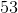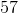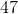# Common Core: 1st Grade Math : Understanding Place Value

## Example Questions

### Example Question #41 : Understanding Place Value

What digit is in the tens place?Explanation:

The tens place is always the second number from the right.

### Example Question #42 : Understanding Place Value

What digit is in the tens place?Explanation:

The tens place is always the second number from the right.

### Example Question #43 : Understanding Place Value

What digit is in the tens place?Explanation:

The tens place is always the second number from the right.

### Example Question #44 : Understanding Place Value

What digit is in the tens place?Explanation:

The tens place is always the second number from the right.

### Example Question #45 : Understanding Place Value

What digit is in the tens place?Explanation:

The tens place is always the second number from the right.

### Example Question #46 : Understanding Place Value

What digit is in the tens place?Explanation:

The tens place is always the second number from the right.

### Example Question #47 : Understanding Place Value

What digit is in the tens place?Explanation:

The tens place is always the second number from the right.

### Example Question #48 : Understanding Place Value

What digit is in the tens place?Explanation:

The tens place is always the second number from the right.

### Example Question #49 : Understanding Place Value

What digit is in the tens place?Explanation:

The tens place is always the second number from the right.

### Example Question #50 : Understanding Place Value

What digit is in the tens place?